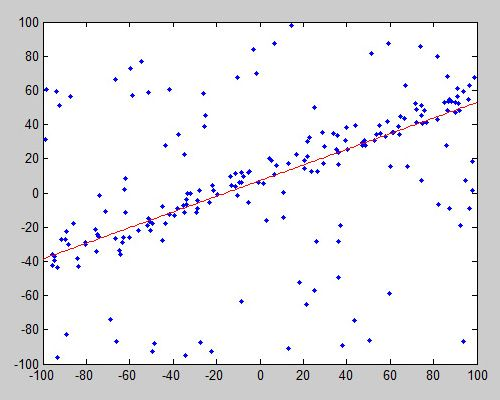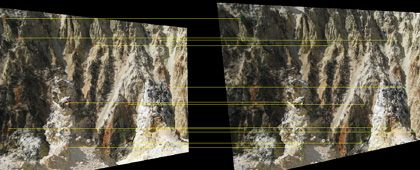RANSAC

Using RANSAC for estimating geometric transforms in computer vision

Random sample consensus, or RANSAC, is an iterative method for estimating a mathematical model from a data set that contains outliers. The RANSAC algorithm works by identifying the outliers in a data set and estimating the desired model using data that does not contain outliers.

RANSAC is accomplished with the following steps

1. Randomly selecting a subset of the data set
2. Fitting a model to the selected subset
3. Determining the number of outliers
4. Repeating steps 1-3 for a prescribed number of iterations

For example, the equation of a line that best fits a set of points can be estimated using RANSAC.Data points shown in blue, with the line of form y = mx+c estimated using RANSAC indicated in red.

In computer vision, RANSAC is used as a robust approach to estimate the fundamental matrix in stereo vision, for finding the commonality between two sets of points for feature-based object detection, and registering sequential video frames for video stabilization.Frames of video stitched together to create a video mosaic. RANSAC is used to estimate the geometric transform between video frames (see example for details).Stereo rectification using feature point matching. RANSAC is used to estimate the fundamental matrix (see example for MATLAB code and explanation).

For details, see Computer Vision Toolbox, which is used with MATLAB and Simulink.

See also: feature extraction, stereo vision, object detection, image recognition, object recognition, RANSAC videos, point cloud